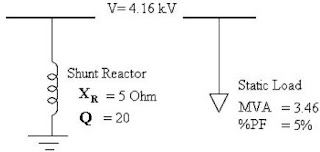## Friday, September 2, 2011

A Shunt Reactor is not yet included in the list of ETAP elements in its Edit Toolbar as of its latest version (ETAP 11). Nonetheless, it doesn’t mean that we can’t model a shunt reactor in our one-line diagram and simulation. Modeling a shunt reactor in ETAP is easy using a simple technique.

The aim of this tutorial is to describe the procedures on how to create a shunt reactor in ETAP using a static load.

1.    To add an element, make sure that you are in Edit Mode. If not, click the Edit button on the Mode toolbar (pencil icon).
2.    Select the static load element on the AC Edit toolbar, and then place it on the One-Line View (OLV). Please refer to the figure below.

4.    Go to the Loading Tab and input the necessary values, which include kV, MVA and PF. ETAP will automatically calculate the MW, Mvar and Amperes.ETAP Static Load Editor
The static load ratings are based from the shunt reactor parameters using the following equations:

Shunt Reactor Parameters

kVR = Reactor Rated Voltage in kV
XR = Reactor Reactance in Ohms
Q = Reactor Quality Factor (X/R)

kVSL = kVR
MVASL = (kVR)2 / XR
PFSL = cos(arctan(Q))Modeling a Shunt Reactor using a Static Load in ETAP
For example:

kVSL = kV = 4.16 kV
MVASL = (kVR)2 / XR  = (4.16)2 / 5
MVASL = 3.46 MVA
PFSL = cos(arctan(Q)) = cos(arctan(20))
PFSL = 5%

5. Select appropriate grounding connection.

6. Click OK.

In this post, modeling a shunt reactor in ETAP was explained using a static load with a rated
power factor determined from the reactor Quality factor.